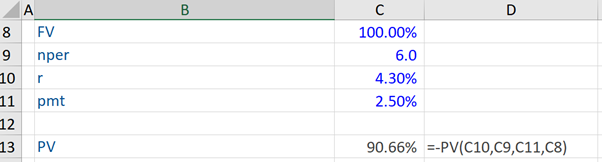## What is “Bond Pricing”?

The term “bond pricing” refers to the formula that is used to calculate the prices of bonds sold in the primary or secondary market. Bonds are priced to yield a certain rate of return to investors and this price should equal the present value of its expected future cash flows. It might be noted that the “coupon rate” of a bond is the rate of interest paid regularly to the bondholder. However, in practice, market practitioners discuss bond yields, which differ from coupons.

In general, the term yield describes the rate of return of a security over a particular period of time and is expressed as a per annum rate. It takes into account coupon income, reinvestment returns, and capital gains/losses investors realize from purchasing a bond.

Having stated the above, bond price plays an important role in the yield of a bond, which is calculated as the annual coupon payment divided by the bond price. Bond prices and yields have an inverse relationship. When bond prices increase (decrease), bond yields decrease (increase).

## Key Learning Points

• Bond pricing plays an important role in relation to bond yields, which is crucial as market practitioners are focused on bond yields.
• In bond pricing, we need to discount all outstanding coupon payments, as well as redemption payment.

## Bond Pricing – Fixed Coupon Bonds

In the case of a fixed coupon bond, cash flows over the life of a bond are known and these payments will occur in the future. Because of the Time Value of Money (TVM) concept, we cannot simply add coupon and redemption payments to get to the bond price. Instead, first, we have to discount all outstanding coupon payments, as well as redemption payment – which is done by dividing each of these payments by 1+r (where r is the desired rate of return per period) to the powers of the relevant number of the period.

Coupon Bond Price = C1/(1+r)^1 + C2/(1+r)^2 +C3/(1+r)^3 + Cn + FVn /(1+r)^n

C= Coupon amount per period (\$)

FV = Face value of the bond (\$)

r = Desired rate of return (%), per period

n = Number of periods to maturity

For example, assume that there is a 10-year fixed coupon bond that pays an annual coupon rate of 3%. If the investor’s desired rate of return is 4% (i.e. rate per period), we have to discount each of the 10 remaining coupon payments and the redemption payment with 1+r(%) – with r=4% – to the power of the period number, which in this case, due to the annual payment frequency, is equal to the number of years (10) until the payment is received.

Bond Price (%) = 3%/(1+4%)^1 +3%/(1+4%)^2 +… (3% + 100%)/(1+4%)^10

Next, suppose there is a 10-year fixed coupon bond that does not pay coupons annually but pays the same semi-annually, the calculation changes. Further, we assume again that the investor’s desired rate of return is 4%. Having stated this, note that the coupon payment per period will not be 3% (as 3% is the coupon rate per annum). As the coupons are paid every six months in this case (i.e. semi-annually), the actual coupon payment per period is 1.5%.

We also need to modify the desired rate we use for discounts. As mentioned in the first example, the desired rate of return used should be the rate per period, but the 4% given here is the “per annum” rate. Assuming that coupons are paid exactly semi-annually for simplicity, the desired rate per period (r) is equal to 2% (which is half of the desired per annum rate of 4%). Further, the total number of periods for a bond with semi-annual payments is going to be double the total number of periods of a bond with annual payments. Therefore, n is equal to 20 here.

Bond Price (%) = 1.5%/(1+2%)^1 +1.5%/(1+2%)^2 + ………… (1.5% + 100%)/(1+2%)^20

## Bond Pricing Example

Assume that an investor’s desired return (r) on a bullet bond is 4.3%. This bond pays an annual coupon rate (pmt) of 2.5% and it matures (nper) in 6 years (no. of periods – nper) i.e. it’s a 6-year bond with annual payments.

Based on this information, we use the PV function in Excel and include r, nper, pmt and the FV (face value) to get a value of 90.66% – which is the calculated bond price.